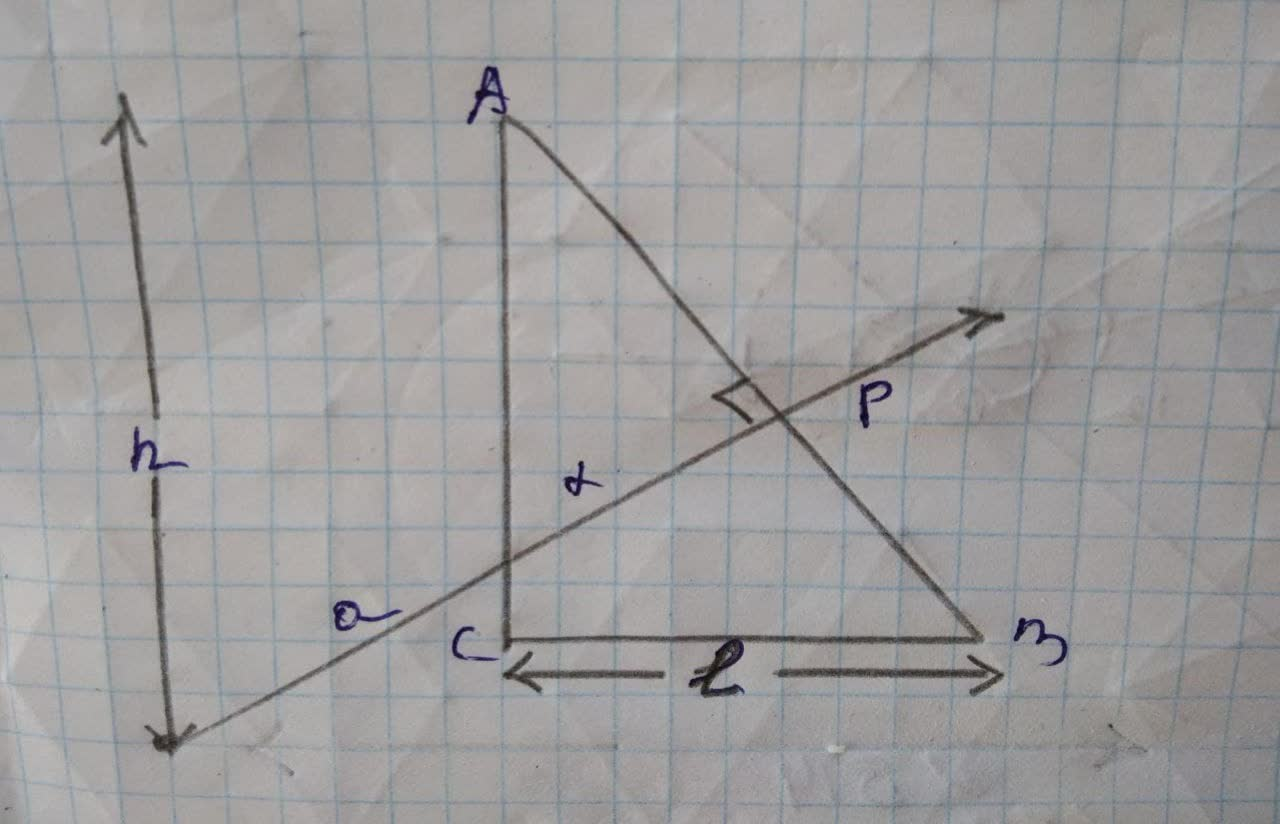## The perpendicular bisector of hypotenuse AB

TrigonometryThe perpendicular bisector of hypotenuse AB2 years ago
The perpendicular bisector of hypotenuse AB in the right triangle △ ABC forms the triangle with the area 3 times smaller than the area of △ ABC. Find the measures of acute angles in △ ABC.(Solve this t without using Trigonometry)
Let the length be CB =l and AC=h in △ ABC.So, by Pythagoras theorem, AB = sqrt(h^(2)+l^(2)) Also, P is a perpendicular bisector of AB. So, AP=PB Hence, AP = PB =(sqrt(h^(2)+l^(2)))/(2) Step2 Here, we see that △ ABC is ~ilar to △ APQ. Since, both are right angle triangles. So, ratio of their sides is (△ ACB)/(△ APQ)=(3)/(1) l=(l)/(sqrt(3))h From the ratio of side one can clearly say that ∠ A = 30°, ∠ B = 60°.